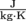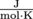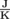## Common Mistakes

### Temperature and Heat

Remember the units! This is true for all of thermodynamics. The constants for latent heat have units and we have to plug those units in along with numbers accordingly. Specific heat, c, for example, is in. If a quiz question gives a temperature change in Fahrenheit, we have to convert them to Kelvin before calculating the heat, Q, that must be supplied to achieve that temperature change. Celsius is a fine unit for temperature changes as well, since a degree Celsius is the same "size" as a degree of Kelvin.

### Thermal Expansion

The three equations for expansion are essentially all the same. If you aren't a walking, talking font of physics knowledge, use the units for area and volume to remember how to convert the coefficients of expansion. Areas have squared units and volumes have cubed units.

To convert the coefficient of linear expansion into the coefficient of area expansion, multiply by 2 (don't square it). Multiply by 3 (no cubing) to get the coefficient of volume expansion.

Okay, you still need to be a spigot of physics knowledge to get this right.

### The Ideal Gas Law

Make a note of the units for the universal gas constant, R. They are. When we use the ideal gas law, all temperatures need to be in Kelvin (unit K).

### Kinetic Theory of Gases

Be careful when converting temperatures, volumes, and masses to the correct units. If the equation involves a constant, such as the Boltzman constant, the units of the constant reveal something about what the units for the rest of the variables should be. The Boltzman constant's units are. That means temperatures should be rockin' it Kelvin-style, not in Celsius.

### The First Law of Thermodynamics

There are two ways to write the first law of thermodynamics. The way we wrote it, ΔU = W + Q, follows a sign convention where work done by the system is negative. Some textbooks will write the first law as ΔU = QW. In this case, work done by the system is positive.

In both cases, heat, Q, is positive when it is put into the system and negative when it leaves the system. Be sure to put in the work to know what kind of work you're doing, or you'll be working overtime to fix your mistakes.

### The Second Law of Thermodynamics and Heat Engines

To calculate efficiencies, we have to use the right sign convention. Work done by the system is negative and work done on the system is positive. Work done by somebody else is one less thing we have to take care of.

### The Carnot Cycle

Review the four steps in the Carnot Cycle as needed, because we definitely wouldn't want to treat an adiabatic process as isothermal, or vice versa. The horror.

### Entropy

Entropy is a measure of disorder. It's not the disorder itself. Furthermore, entropy doesn't mean that a system can't become ordered, just that a system can't order itself. Ordering the system requires added energy from elsewhere, such as all that elbow grease we used to clean our room.